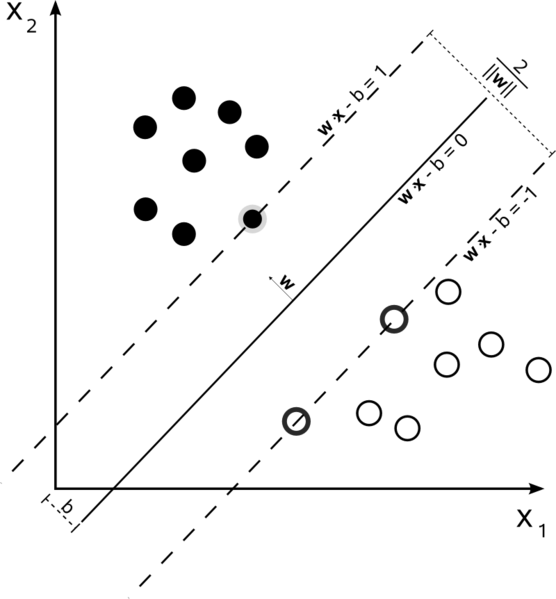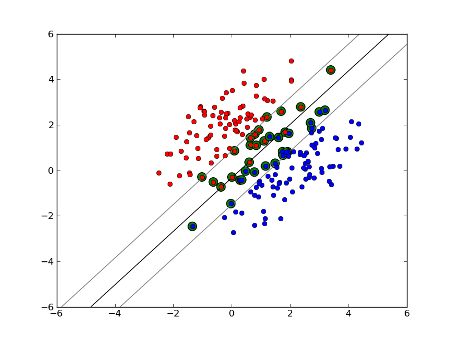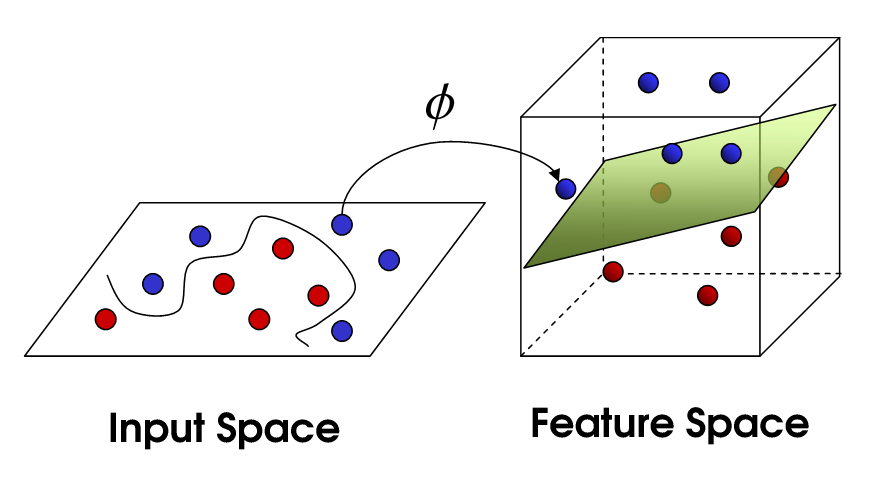2 views

I am using LibSVM to classify some documents. The documents seem to be a bit difficult to classify as the final results show. However, I have noticed something while training my models. and that is: If my training set is for example 1000 around 800 of them are selected as support vectors. I have looked everywhere to find if this is a good thing or bad. I mean is there a relation between the number of support vectors and the performance of the classifier? I have read this post previous post. However, I am performing a parameter selection and also I am sure that the attributes in the feature vectors are all ordered. I just need to know the relation. Thanks. p.s: I use a linear kernel.

by (33.1k points)

Support Vector Machines: SVM is a commonly used machine learning algorithm. It works on the principle to find a hyperplane that divides the two classes with the largest margin.most of the data points which fall within this margin.

It performs classification in the following ways:

Hard Margin Linear SVM

If data is linearly separable, then you can use a hard margin SVM classifier, the support vectors in this technique are the points which lie along the supporting hyperplanes.Almost every support vectors lie exactly on the margin. Support vectors are independent of the number of dimensions or size of the data set, the number of support vectors can be at least two.

Soft-Margin Linear SVM

This technique is used when data is non- linearly separable. It is not required that our data points lie outside the margin. There is a slack parameter C used to control this. This gives us a larger margin and greater error on the training dataset, but improves generalization and/or allows us to find a linear separation of data that is not linearly separable.Non-Linear SVM

We use different kernel functions in SVM. They have their own set of parameters. When we translate this back to the original feature space, the result is non-linear: